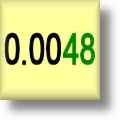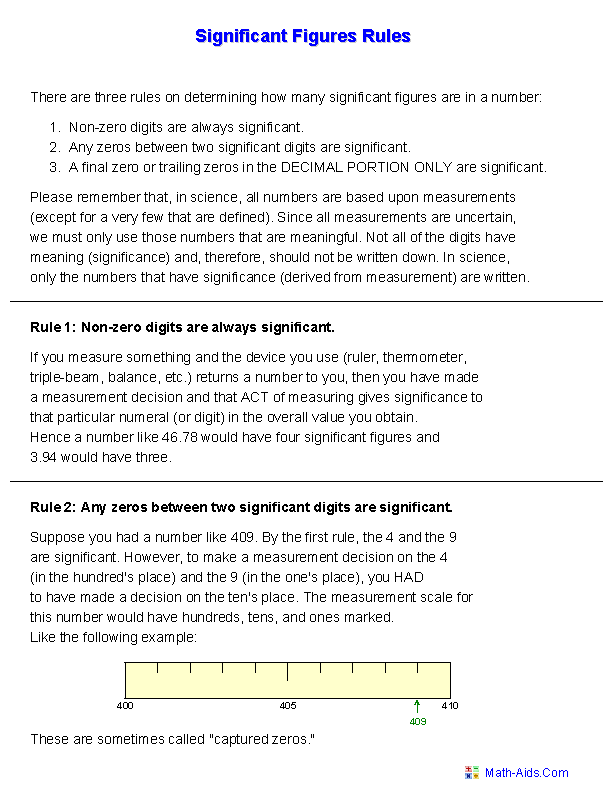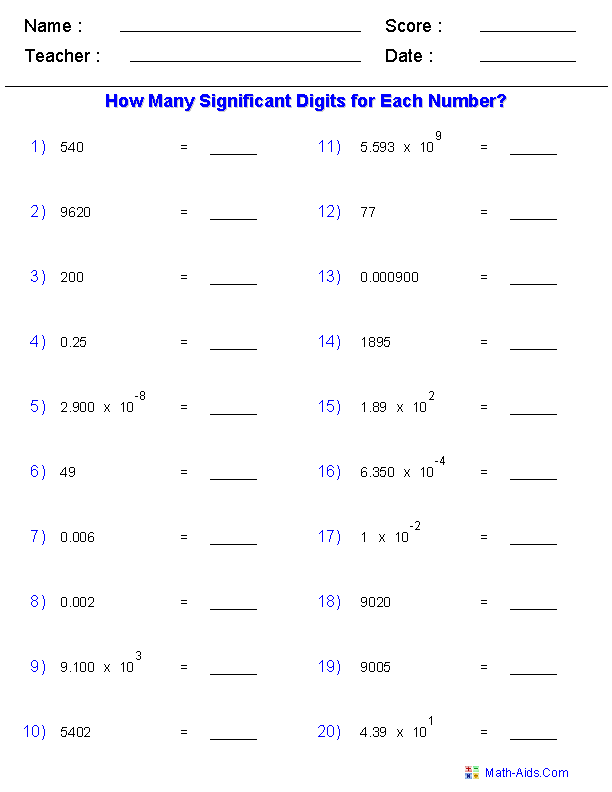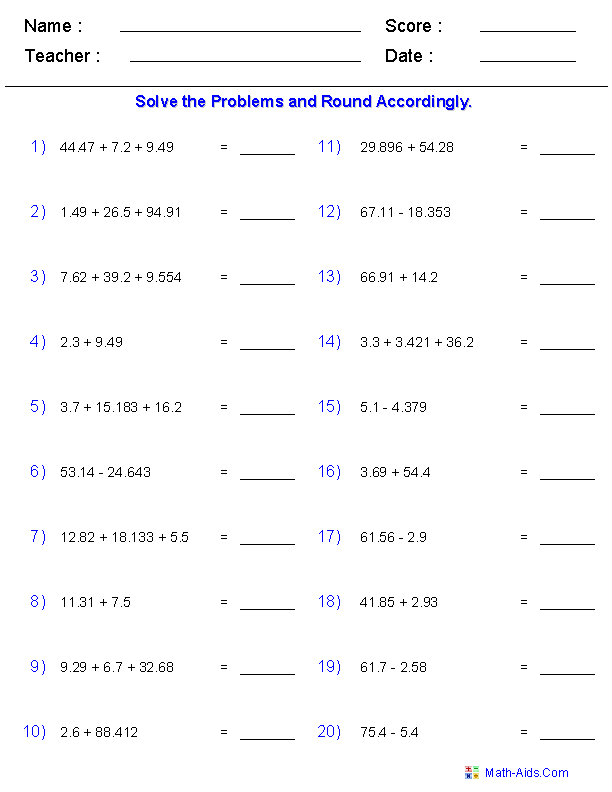#Significant Figures Worksheets

## Printable Significant Figures Worksheets

These Significant Figures Worksheets are great for testing students in their ability on identifying and working with significant digits. The "Significant Figures Rules Handout Worksheet" is great for reinforcing the rules in determining the correct number of significant digits in a number.

Use the "Identify Significant Digits Worksheet" to measure their ability to correctly identify the number of significant digits in a number.

The "Adding and Subtracting" and Multiplying and Dividing" with Significant Figures Worksheets are great for solving problems with significant digits and rounding to the correct answer.

Click here for a Detailed Description of all the Significant Figures Worksheets.

## Quick Link for All Significant Figures Worksheets

Click the image to be taken to that Significant Figures Worksheet.

#### Significant FiguresRules Handout#### Identify Significant DigitsSignificant Figures#### Adding and Subtractingwith Significant Figures#### Multiplying and Dividingwith Significant FiguresRecommended Videos

## Detailed Description for All Significant Figures Worksheets

Significant Figures Rules Handout
This Significant Figures Worksheet is a great handout for reinforcing the rules of significant figures.

Identify Significant Digits Worksheet
These Significant Figures Worksheets are great for testing the students in their ability to determine the number of significant digits for a given number. You may select the numbers to be whole, decimal, scientific notation, or all three. These Significant Figures Worksheets will produce twenty problems per worksheet.

Adding and Subtracting with Significant Figures Worksheet
These Significant Figures Worksheets are great for solving addition and subtraction problems with significant figures and correctly rounding to the correct answer. You may select the problems to be addition, subtraction, or both. These Significant Figures Worksheets will produce twenty problems per worksheet.

Multiplying and Dividing with Significant Figures Worksheet
These Significant Figures Worksheets are great for solving multiplication and division problems with significant figures and correctly rounding to the correct answer. You may select the problems to be multiplication, division, or both. These Significant Figures Worksheets will produce twenty problems per worksheet.